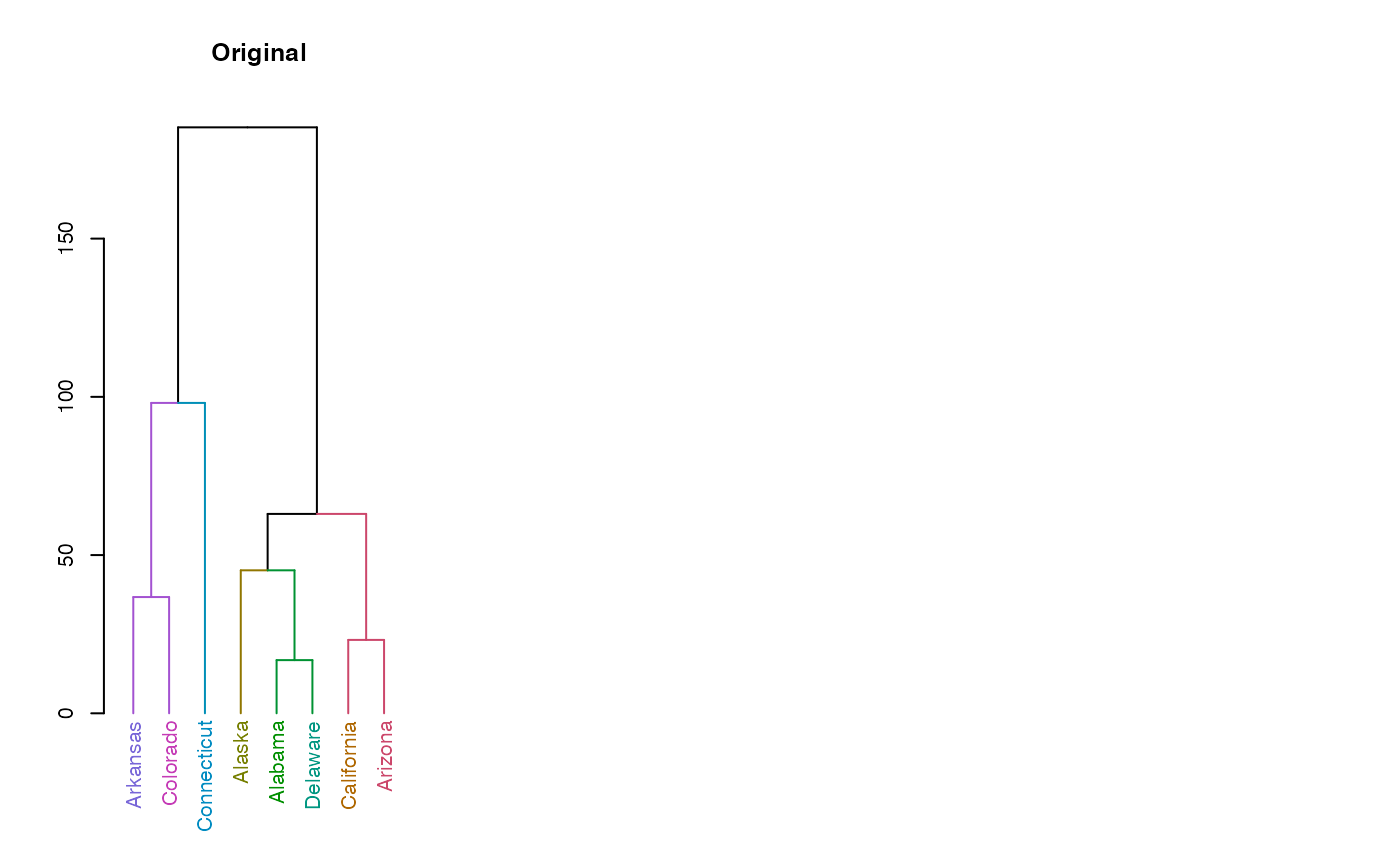This function reorganizes the internal structure of the tree to get the ladderized effect when plotted.

ladderize(x, right = TRUE, ...)

# S3 method for dendrogram

# S3 method for phylo
ladderize(x, right = TRUE, phy, ...)

# S3 method for dendlist
ladderize(x, right = TRUE, which, ...)

## Arguments

x

a tree object (either a dendrogram, dendlist, or phylo)

right

a logical (TRUE) specifying whether the smallest clade is on the right-hand side (when the tree is plotted upwards), or the opposite (if FALSE).

...

Currently ignored.

phy

a placeholder in case the user uses "phy ="

which

an integer (can have any number of elements). It indicates the elements in the dendlist to ladderize. If missing, it will ladderize all the dendrograms in the dendlist.

## Value

A rotated tree object

ladderize, rev.dendrogram, rotate (dendextend), rotate (ape)

## Examples


dend <- USArrests[1:8, ] %>%
dist() %>%
hclust() %>%
as.dendrogram() %>%
set("labels_colors") %>%
set("branches_k_color", k = 5)
set.seed(123)
dend <- shuffle(dend)

par(mfrow = c(1, 3))
dend %>% plot(main = "Original")
dend %>%